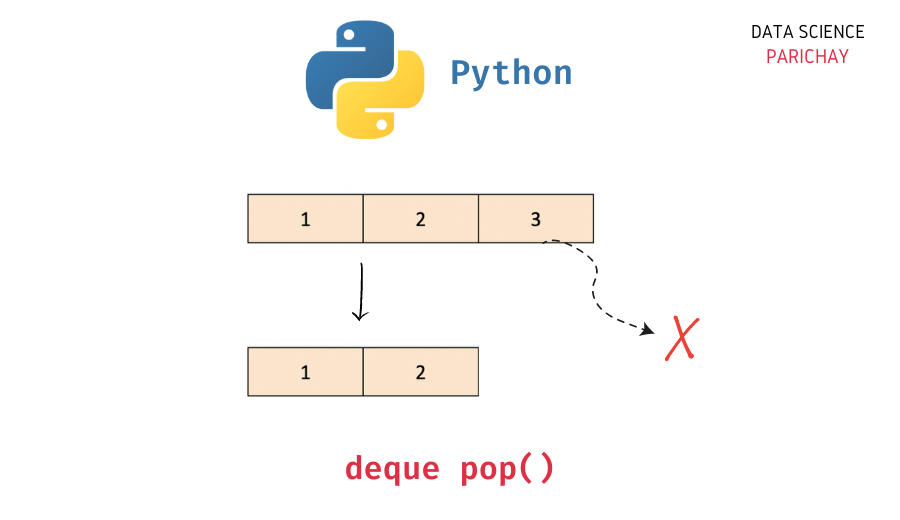# Python – Pop Element From the End in Deque

In this tutorial, we will look at how to pop an element from the end of a deque in Python with the help of some examples.

## `deque` in Python

The `collections` module in Python comes with a `deque` class that you can use to implement a stack or queue data structure in Python. Deques support thread-safe, memory efficient appends and pops from either side of the deque with approximately the same O(1) performance in either direction.

In simple terms, you can think of a `deque` in Python as a list-like data structure but with efficient insertion and removal of items from either end (left and right). This makes them very useful when implementing a stack or queue functionality in Python.

## How to pop an element from the end of a deque in Python?

The `deque` class comes with a number of built-in functions to help perform common operations (like adding and removing elements).

To pop (remove) an element from the end of a deque in Python, use the `pop()` function. The following is the syntax –

```# pop the right most element from deque, "queue"
queue.pop()```

It removes the last (rightmost) element from the deque and returns it. If no elements are present in the deque, it raises an `IndexError`.

## Examples

Let’s now look at some examples of using the above syntax –

### Example 1 – Pop element from the end of a non-empty deque

First, let’s create a deque with some elements.

📚 Data Science Programs By Skill Level

Introductory

Intermediate ⭐⭐⭐

🔎 Find Data Science Programs 👨‍💻 111,889 already enrolled

Disclaimer: Data Science Parichay is reader supported. When you purchase a course through a link on this site, we may earn a small commission at no additional cost to you. Earned commissions help support this website and its team of writers.

```from collections import deque

# create a deque
queue = deque([1, 2, 3])
# print the deque
print(queue)```

Output:

`deque([1, 2, 3])`

Here, we imported the `deque` class from the `collections` module and created a deque object, `queue` with three elements – 1, 2, and 3.

Now, let’s pop an element from the end (right side) of this deque object.

```# pop the last (rightmost) element
val = queue.pop()
# print the poppend element
print(val)
# print the deque
print(queue)```

Output:

```3
deque([1, 2])```

Here, we used the `pop()` function to pop-out (remove) the last value in the deque, `queue`. You can see that it removes the rightmost value and returns it.

### Example 2 – Pop element from the end of an empty deque

Let’s now create an empty deque object (a deque with no elements).

```# create an empty deque
queue = deque()
# print the deque
print(queue)```

Output:

`deque([])`

Now, let’s try to remove an element from the end of this deque using the `pop()` function.

```# pop the last (rightmost) element
val = queue.pop()
# print the poppend element
print(val)
# print the deque
print(queue)```

Output:

```---------------------------------------------------------------------------
IndexError                                Traceback (most recent call last)
Input In , in <module>
1 # pop the last (rightmost) element
----> 2 val = queue.pop()
3 # print the poppend element
4 print(val)

IndexError: pop from an empty deque```

You can see that we get an `IndexError`. This happened because we are trying to remove an element from an empty deque object (which does not have any elements).

## Difference between `popleft()` and `pop()`

Both the `popleft()` and the `pop()` functions are used to remove an element from a deque in Python. The main difference between them is that the `pop()` function is used to remove an element from the end (the right side) of the deque whereas the `popleft()` function is used to remove an element from the start (the left side) of the deque.

You might also be interested in –

•